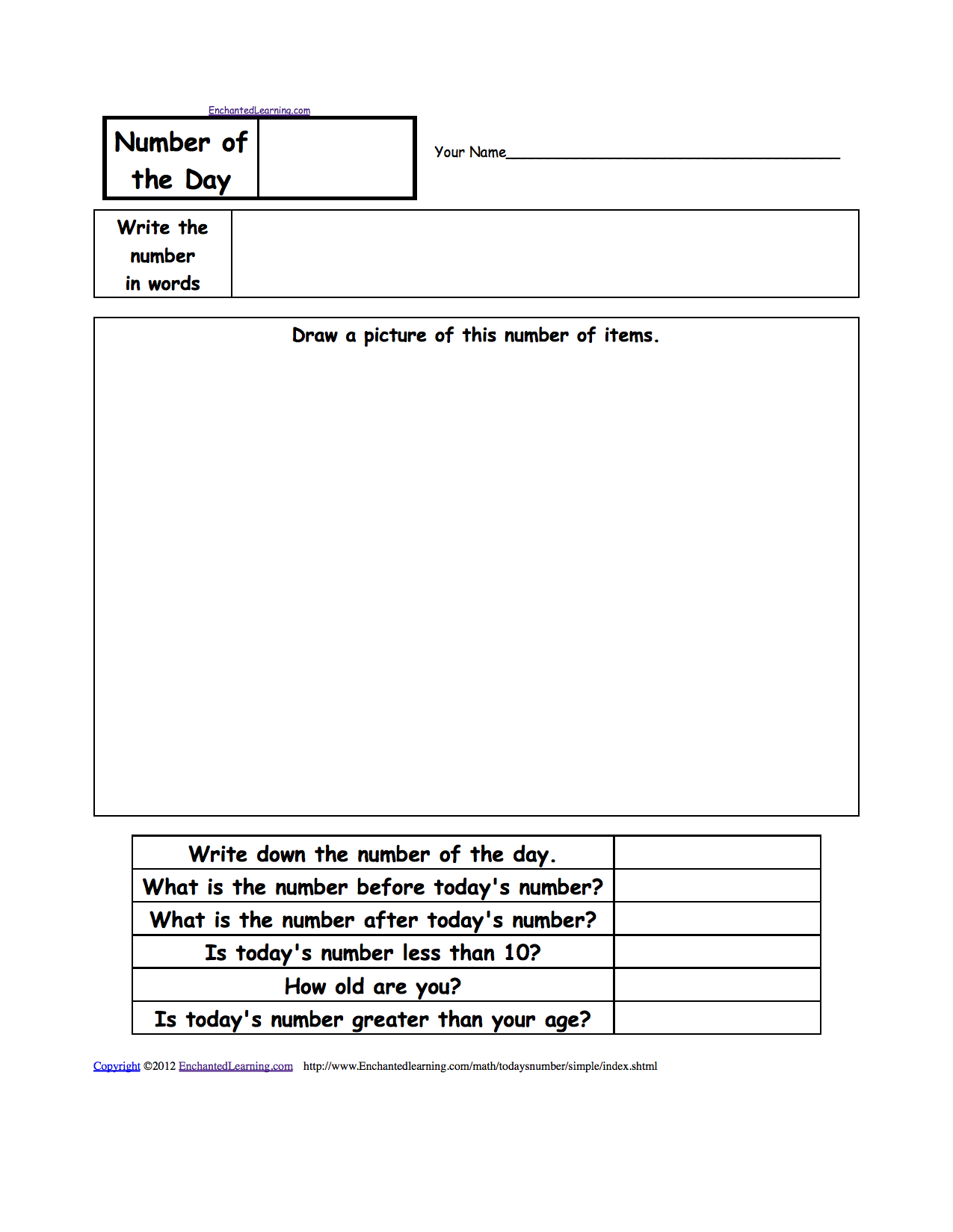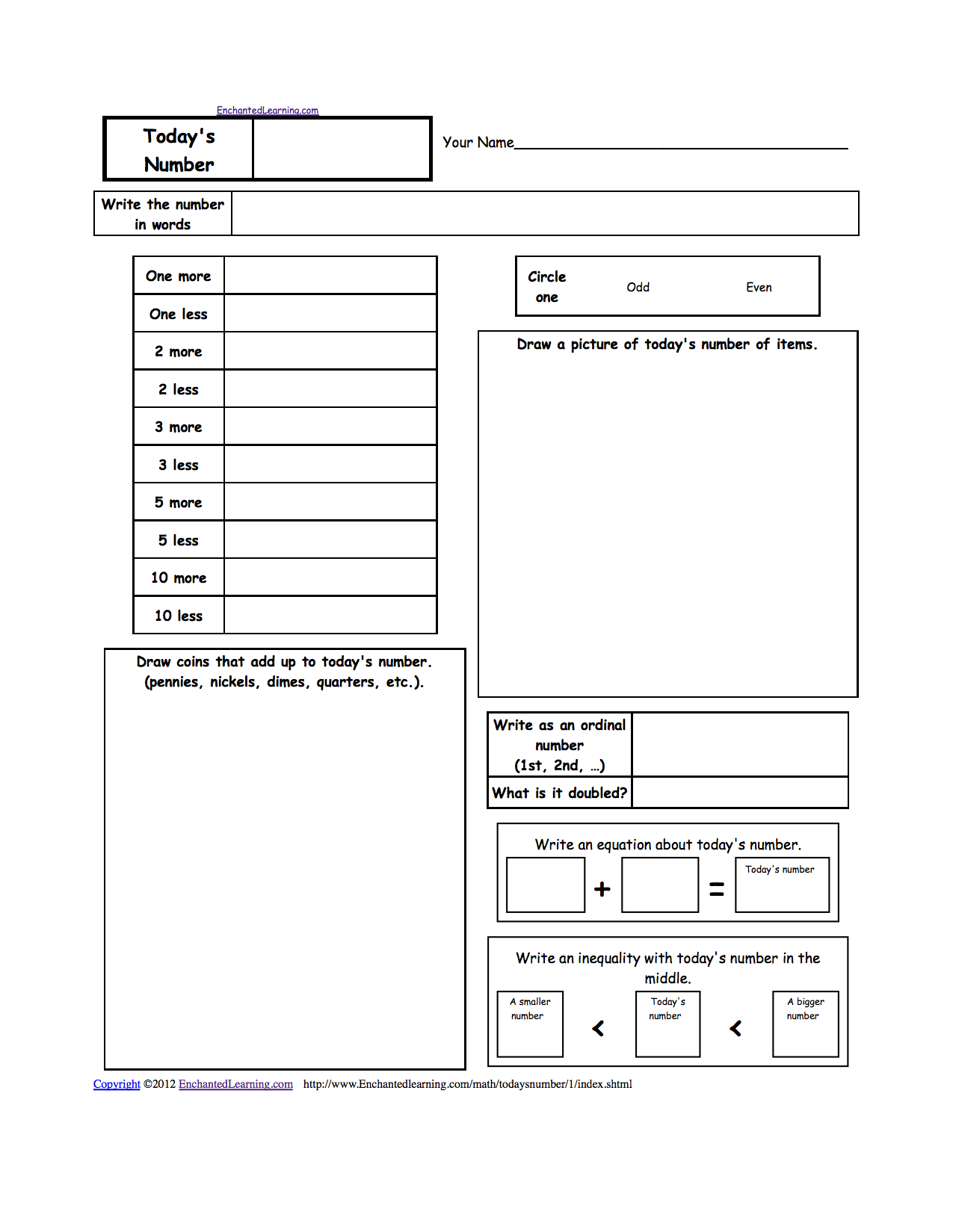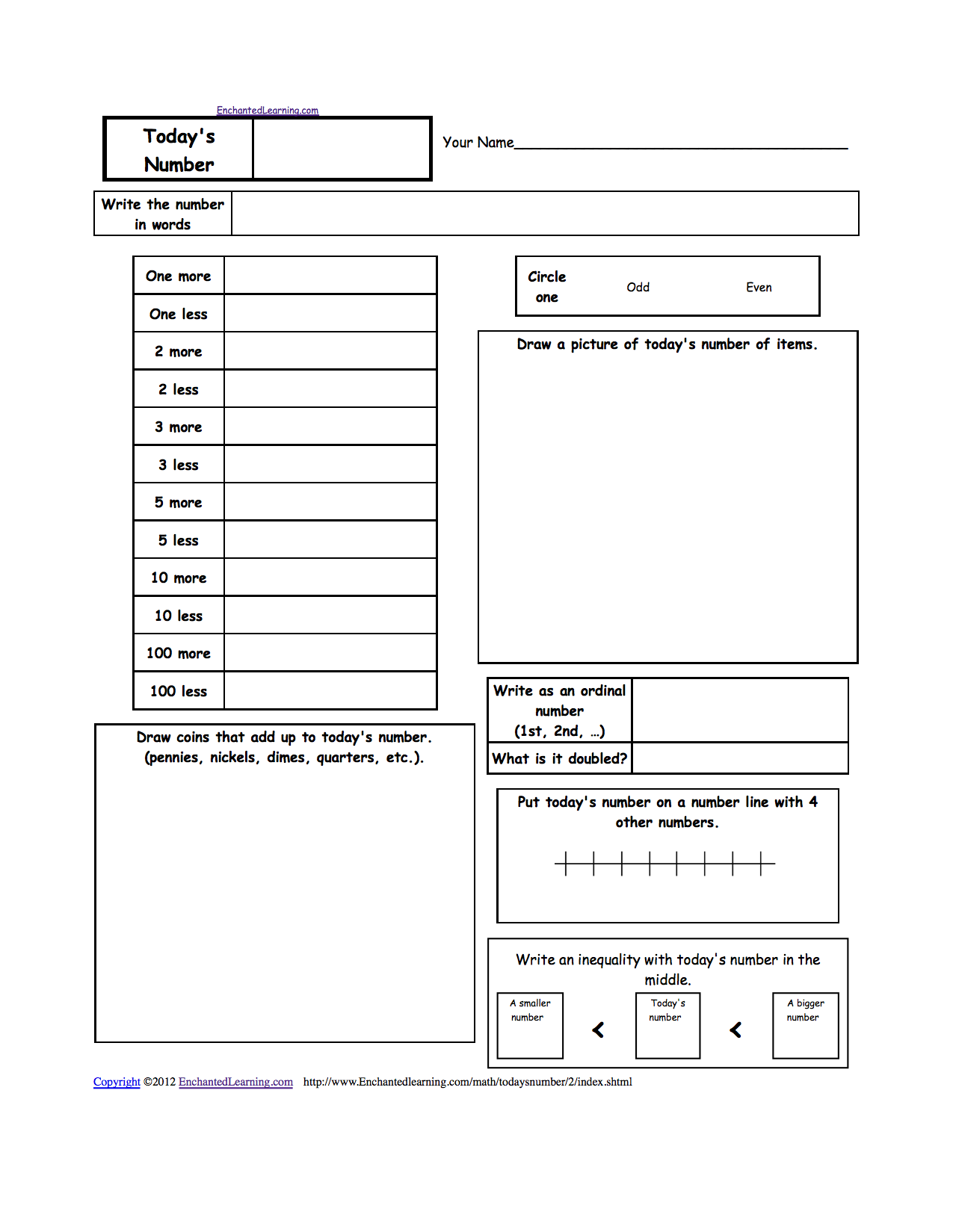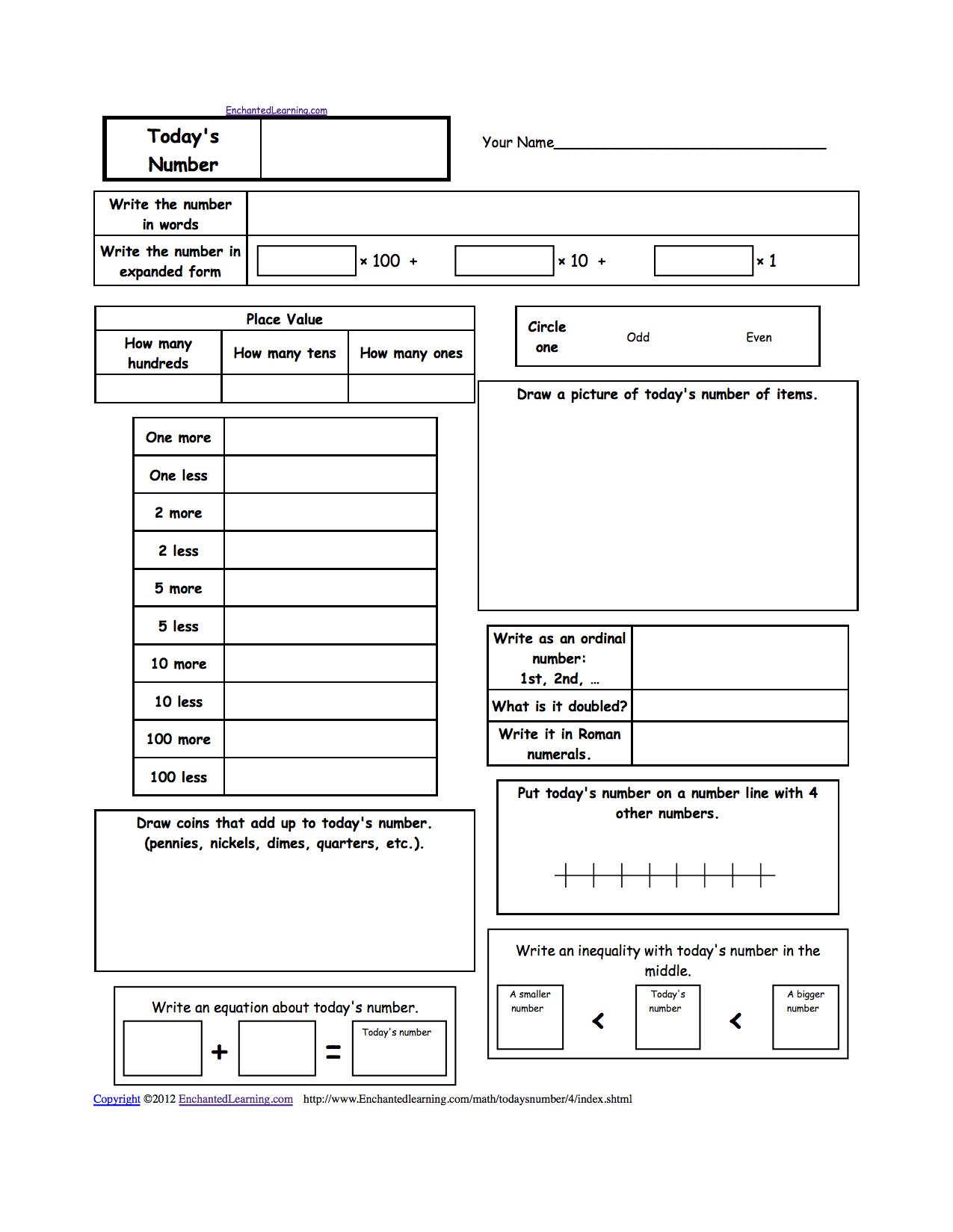EnchantedLearning.com is a user-supported site.
As a bonus, site members have access to a banner-ad-free version of the site, with print-friendly pages.

 Number of the Day Worksheets Math
These worksheets on elementary math let the student do an in-depth examination of a number that the teacher supplies. The student writes the number of the day in words, draws it in everyday objects, answers simple questions about the number, writes it in expanded form, determines if it is even or odd, adds and subtracts one, two, ten, etc., draws it in coins, writes it in Roman numerals, puts it on a number line, puts it in an equation, writes an inequality about it, etc.

Worksheets on Exploring Numbers Each Day:Number of the Day - Simplest VersionThis worksheet on elementary math lets the student do an in-depth examination of a number that the teacher supplies. The student writes the number in words, draws it in everyday objects, and answers a few simple questions about the number (for example, "Is today's number greater than your age?"). Or go to a pdf of the worksheet (subscribers only).Today's Number - Easy Version #1This worksheet on elementary math lets the student do an in-depth examination of a number that the teacher supplies. The student writes the number in words, determines if it is even or odd, adds and subtracts one, two, ten, etc., draws it in coins, draws it in everyday objects, writes an equation with the number, etc. Or go to a pdf of the worksheet (subscribers only).Today's Number - Easy Version #2This worksheet on elementary math lets the student do an in-depth examination of a number that the teacher supplies. The student writes the number in words, determines if it is even or odd, adds and subtracts one, two, ten, etc., draws it in coins, draws it in everyday objects, puts it on a number line, etc. Or go to a pdf of the worksheet (subscribers only).Today's NumberThis worksheet on elementary math lets the student do an in-depth examination of a number that the teacher supplies. The student writes the number in words, writes it in expanded form, determines if it is even or odd, adds and subtracts one, two, ten, etc., draws it in coins, draws it in everyday objects, writes it in Roman numerals, puts it on a number line, etc. Or go to a pdf of the worksheet (subscribers only).Today's Date (as a number)This worksheet on elementary math lets the student do an in-depth examination of the mathematics of the day of the month (a number between 1 and 31). The student writes the date in words, writes it in expanded form, determines if it is even or odd, adds and subtracts one, two, ten, etc., draws it in coins, draws it in everyday objects, writes it in Roman numerals, puts it on a number line, etc. Or go to a pdf of the worksheet (subscribers only).The Number One HundredThis worksheet on elementary math lets the student do an in-depth examination of the number 100. The student writes the number in words, determines if it is even or odd, adds and subtracts one, two, ten, etc., draws it in coins, draws it in everyday objects, writes it in Roman numerals, halves it, doubles it, puts it on a number line, etc. Or go to the answers. Or go to a pdf of the worksheet plus the answers (subscribers only).

 +, - EnchantedLearning.comMath x, ÷
 A B C D E F G H I J K L M N O P Q R S T U V W X Y Z
 Number Line Fractions Decimals Measurement Rounding Graphs

Enchanted Learning®
Over 35,000 Web Pages
Sample Pages for Prospective Subscribers, or click below

 Overview of Site What's New Enchanted Learning Home Monthly Activity Calendar Books to Print Site Index K-3 Crafts K-3 Themes Little ExplorersPicture dictionary PreK/K Activities Rebus Rhymes Stories Writing Cloze Activities Essay Topics Newspaper Writing Activities Parts of Speech Fiction The Test of Time Biology Animal Printouts Biology Label Printouts Biomes Birds Butterflies Dinosaurs Food Chain Human Anatomy Mammals Plants Rainforests Sharks Whales Physical Sciences: K-12 Astronomy The Earth Geology Hurricanes Landforms Oceans Tsunami Volcano Languages Dutch French German Italian Japanese (Romaji) Portuguese Spanish Swedish Geography/History Explorers Flags Geography Inventors US History Other Topics Art and Artists Calendars College Finder Crafts Graphic Organizers Label Me! Printouts Math Music Word Wheels

## Enchanted Learning Search

 Search the Enchanted Learning website for: